﻿ 圆型限制性三体问题中转移轨道类型的数值研究 Numerical Study on the Species of Transfer Orbit in the Circular Restricted Three-Body Problem

Astronomy and Astrophysics
Vol.06 No.01(2018), Article ID:23371,10 pages
10.12677/AAS.2018.61001

Numerical Study on the Species of Transfer Orbit in the Circular Restricted Three-Body Problem

Yu Huang, Fan Lin, Yiqing Shen, Ruifang Wang, Fabao Gao*

College of Mathematical Science, Yangzhou University, Yangzhou Jiangsu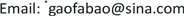Received: Dec. 19th, 2017; accepted: Jan. 3rd, 2018; published: Jan. 10th, 2018ABSTRACT

Based on the dynamical equations of spatial circular restricted three-body problem, the bifurcation diagram of the system with the mass ratio as the bifurcation parameter is demonstrated, and it is found that when the masses of the two main bodies are considerable equivalent (i.e., the mass ratio is around 0.5), the third body’s dynamic behavior is more complicated than at other mass ratios. When the mass ratio is in the interval (0.4, 0.6), we further simulate 32,000 transfer orbits and make a brief classification of the found orbits.

Keywords:CR3BP, Bifurcation Diagram, Transfer Orbit, Interplanetary Interchange Station1. 引言

2. 限制性三体系统建模及分析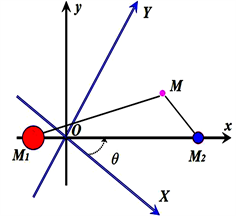Figure 1. Coordinate system

$\begin{array}{l}{\stackrel{˙}{x}}_{1}={x}_{4},\\ {\stackrel{˙}{x}}_{2}={x}_{5},\\ {\stackrel{˙}{x}}_{3}={x}_{6},\\ {\stackrel{˙}{x}}_{4}={x}_{1}+2{x}_{5}-\frac{\left(1-\mu \right)\left({x}_{1}+\mu \right)}{{\left[{\left({x}_{1}+\mu \right)}^{2}+{x}_{2}^{2}+{x}_{3}^{2}\right]}^{\frac{3}{2}}}-\frac{\mu \left({x}_{1}-1+\mu \right)}{{\left[{\left({x}_{1}-1+\mu \right)}^{2}+{x}_{2}^{2}+{x}_{3}^{2}\right]}^{\frac{3}{2}}},\\ {\stackrel{˙}{x}}_{5}={x}_{2}-2{x}_{4}-\frac{\left(1-\mu \right){x}_{2}}{{\left[{\left({x}_{1}+\mu \right)}^{2}+{x}_{2}^{2}+{x}_{3}^{2}\right]}^{\frac{3}{2}}}-\frac{\mu {x}_{2}}{{\left[{\left({x}_{1}-1+\mu \right)}^{2}+{x}_{2}^{2}+{x}_{3}^{2}\right]}^{\frac{3}{2}}},\\ {\stackrel{˙}{x}}_{6}=-\frac{\left(1-\mu \right){x}_{3}}{{\left[{\left({x}_{1}+\mu \right)}^{2}+{x}_{2}^{2}+{x}_{3}^{2}\right]}^{\frac{3}{2}}}+\frac{\mu {x}_{3}}{{\left[{\left({x}_{1}-1+\mu \right)}^{2}+{x}_{2}^{2}+{x}_{3}^{2}\right]}^{\frac{3}{2}}}.\end{array}$

3. 系统的分岔图及转移轨道的数值研究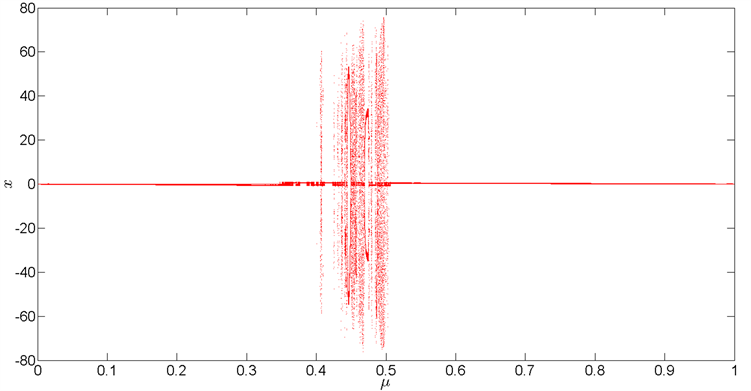(a)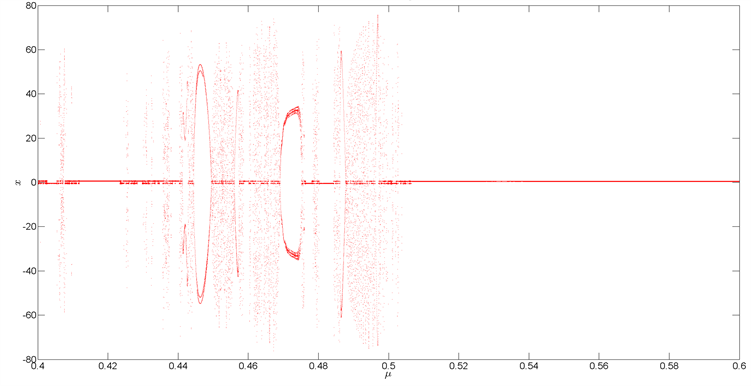(b)

Figure 2. (a) Bifurcation diagram for $\mu \in \left(0,1\right)$ ; (b) Bifurcation diagram for $\mu \in \left(0.4,0.6\right)$

4. 结论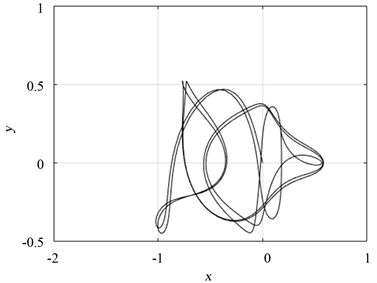Figure 3. Mass ratio of $\mu =0.43559$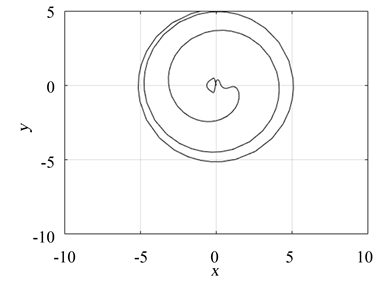Figure 4. Mass ratio of $\mu =0.45859$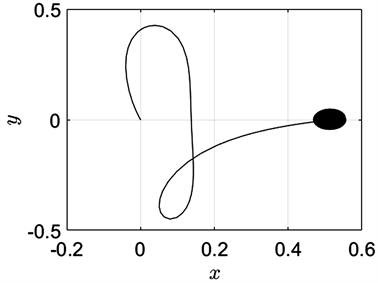Figure 5. Mass ratio of $\mu =0.48505$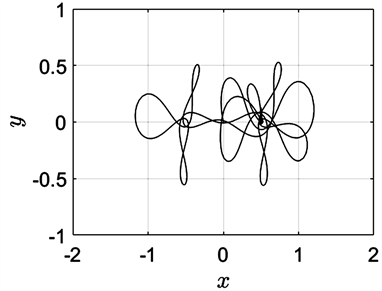Figure 6. Mass ratio of $\mu =0.49999$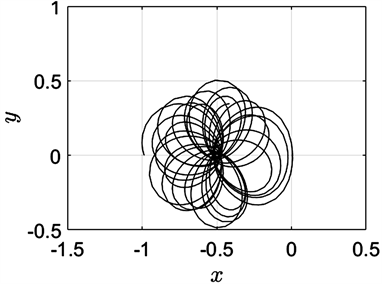Figure 7. The initial value in the X direction is −0.986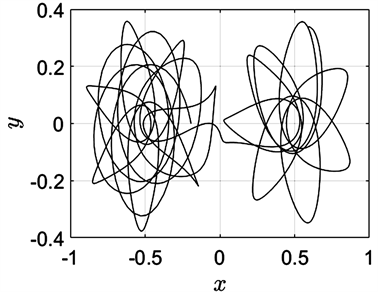Figure 8. The initial value in the X direction is −0.193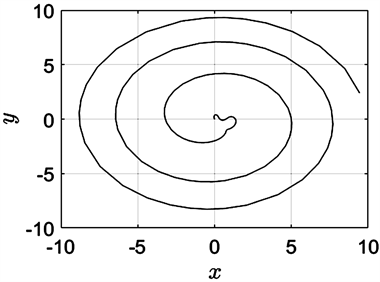Figure 9. The initial value in the X direction is −0.004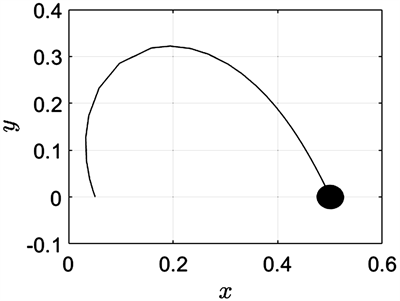Figure 10. The initial value in the X direction is 0.051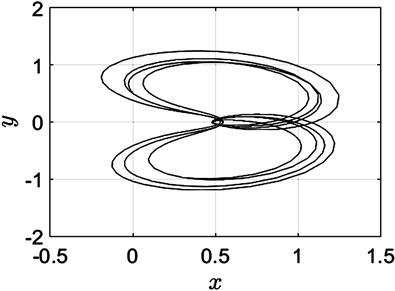Figure 11. The initial value in the Y direction is 0.515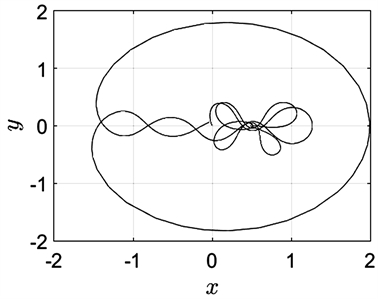Figure 12. The initial value in the Z direction is −0.107Figure 13. The initial velocity in the X direction is −0.48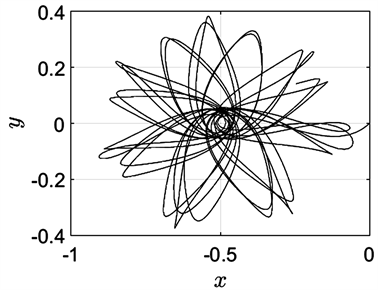Figure 14. The initial velocity in the Y direction is −0.3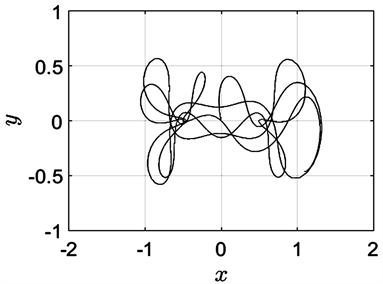Figure 15. The initial velocity in the Z direction is −0.44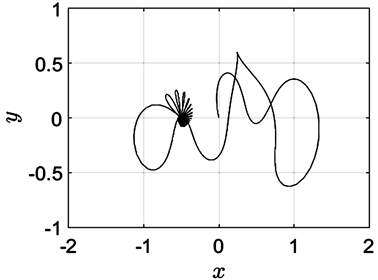Figure 16. The initial velocity in the Z direction is −0.5

Numerical Study on the Species of Transfer Orbit in the Circular Restricted Three-Body Problem[J]. 天文与天体物理, 2018, 06(01): 1-10. http://dx.doi.org/10.12677/AAS.2018.61001

1. 1. 刘暾, 赵钧. 空间飞行器动力学[M]. 哈尔滨: 哈尔滨工业大学出版社, 2003.

2. 2. Curtis, H.D. (2005) Orbital Mechanics for Engineering Students. Elsevier, Amsterdam.

3. 3. 刘林, 侯锡云. 深空探测器轨道力学[M]. 北京: 电子工业出版社, 2012.

4. 4. Broucke, R.A. (1968) Periodic Orbits in the Restricted Three-Body Problem with Earth-Moon Masses, Jet Propulsion Laboratory. Technical Report, 82-1168.

5. 5. Chenciner, A. and Montgomery, R. (2000) A Remarkable Periodic Solution of the Three Body Problem in the Case of Equal Masses. Annals of Mathematics, 152, 881-901.
https://doi.org/10.2307/2661357

6. 6. Šuvakov, M. and Dmitrašinović, V. (2013) Three Classes of Newtonian Three-Body Planar Periodic Orbits. Physical Review Letters, 110, 114301.
https://doi.org/10.1103/PhysRevLett.110.114301

7. 7. Crane, L. (2017) Three-Body Problem Gets 1000 Solutions. NewScientist, 235, 14 p.
https://doi.org/10.1016/S0262-4079(17)31908-5

8. 8. Li, X.M., Jing, Y.P. and Liao, S.J. (2017) The 1223 New Periodic Orbits of Planar Three-Body Problem with Unequal Mass and Zero Angular Momentum. arXiv:1709.04775v1

9. 9. Li, X.M. and Liao, S.J. (2017) More Than Six Hundred New Families of Newtonian Periodic Planar Collisionless Three-Body Orbits. Science China Physics, Mechanics & Astronomy, 60, 129511.
https://doi.org/10.1007/s11433-017-9078-5

10. 10. 高发宝. 深空探测中非线性动力学及周期轨道的研究[D]: [博士学位论文]. 北京: 北京工业大学, 2012.

11. NOTES



*通讯作者。